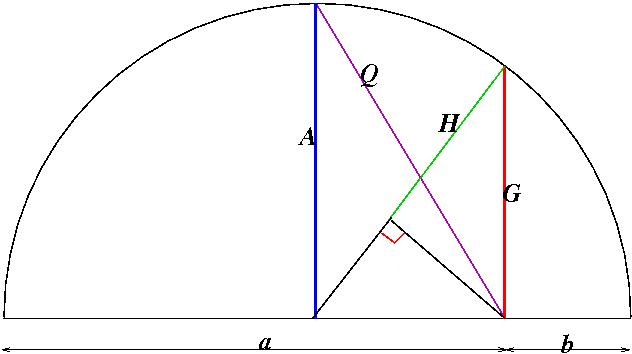#### You may also like### Shades of Fermat's Last Theorem

The familiar Pythagorean 3-4-5 triple gives one solution to (x-1)^n + x^n = (x+1)^n so what about other solutions for x an integer and n= 2, 3, 4 or 5?### Exhaustion

Find the positive integer solutions of the equation (1+1/a)(1+1/b)(1+1/c) = 2### Code to Zero

Find all 3 digit numbers such that by adding the first digit, the square of the second and the cube of the third you get the original number, for example 1 + 3^2 + 5^3 = 135.

# Classical Means

##### Age 16 to 18 Challenge Level:

 Why do this problem?Doing this problem you need to visualise the relationship between the formulae for the arithmetic, geometric and harmonic means in terms of the radius of a circle and the geometry of similar triangles and Pythagoras Theorem. This process involves a beautiful blend of algebra and geometry. It provides a good exercise for learners in mathematical reasoning and proof. The mathematical concepts are simple and the interplay of ideas to give the necessary proofs is good experience for learners.
Possible approach
Ask the learners to work out the radius of the semicircle in terms of $a$ and $b$. Then ask them to identify all the triangles and to discuss with a partner, and write down, everything they observe about the triangles. They may then be able to do the problem for themselves. If not the following questions should provide some assistance.
Key questions
Can you find the radius of the semicircle?
Can you see a right-angled triangle with $G$ as the length of one side and use this to find $G$ in terms of $a$ and $b$?
Can you use similar triangles to find a relationship between $A$, $G$ and $H$?
Can you use similar triangles to find a formula for $H$ in terms of $a$ and $b$?
Can you you use the geometry of triangles to compare the lengths of $A$, $G$ and $H$?
Can you see a right-angled triangle with $Q$ as the length of one side and use this to find $Q$ in terms of $a$ and $b$?

Possible extension
Try the problem Pythagorean Golden Means and read the article About Pythagorean Golden Means

Possible support
Try the problem Harmonic Triangle.